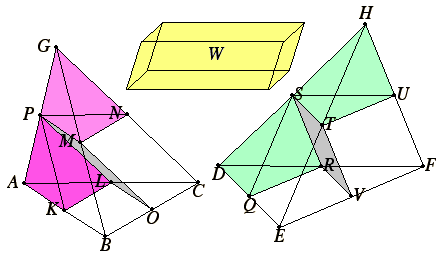# Proposition 5

Pyramids of the same height with triangular bases are to one another as their bases.

Let there be pyramids of the same height with triangular bases ABC and DEF and vertices G and H.

I say that the base ABC is to the base DEF as the pyramid ABCG is to the pyramid DEFH.

For, if the pyramid ABCG is not to the pyramid DEFH as the base ABC is to the base DEF, then the base ABC is to the base DEF as the pyramid ABCG is either to some solid less than the pyramid DEFH or to a greater solid.Let it, first, be in that ratio to a less solid W.

Divide the pyramid DEFH into two pyramids equal to one another and similar to the whole and into two equal prisms.

XII.3

Then the two prisms are greater than the half of the whole pyramid.

X.1

Again, divide the pyramids arising from the division similarly, and let this be done repeatedly until there are left over from the pyramid DEFH some pyramids which are less than the excess by which the pyramid DEFH exceeds the solid W.

Let such be left, and let them be, for the sake of argument, DQRS and STUH. Therefore the remainders, the prisms in the pyramid DEFH, are greater than the solid W.

XII.4

Divide the pyramid ABCG similarly, and a same number of times, with the pyramid DEFH. Therefore the base ABC is to the base DEF as the prisms in the pyramid ABCG are to the prisms in the pyramid DEFH.

But the base ABC is to the base DEF as the pyramid ABCG is to the solid W, therefore the pyramid ABCG is to the solid W as the prisms in the pyramid ABCG are to the prisms in the pyramid DEFH. Therefore, alternately the pyramid ABCG is to the prisms in it as the solid W is to the prisms in the pyramid DEFH.

But the pyramid ABCG is greater than the prisms in it, therefore the solid W is also greater than the prisms in the pyramid DEFH.

But it is also less, which is impossible.

Therefore the prism ABCG is not to any solid less than the pyramid DEFH as the base ABC is to the base DEF.

Similarly it can be proved that neither is the pyramid DEFH to any solid less than the pyramid ABCG as the base DEF is to the base ABC.

I say next that neither is the pyramid ABCG to any solid greater than the pyramid DEFH as the base ABC is to the base DEF.

For, if possible, let it be in that ratio to a greater solid W.

Therefore, inversely the base DEF is to the base ABC as the solid W is to the pyramid ABCG.

But it was proved before that the solid W is to the solid ABCG as the pyramid DEFH is to some solid less than the pyramid ABCG. Therefore the base DEF is to the base ABC as the pyramid DEFH is to some solid less than the pyramid ABCG, which was proved absurd.

Therefore the pyramid ABCG is not to any solid greater than the pyramid DEFH as the base ABC is to the base DEF.

But it was proved that neither is it in that ratio to a less solid.

Therefore the base ABC is to the base DEF as the pyramid ABCG is to the pyramid DEFH.

Therefore, pyramids of the same height with triangular bases are to one another as their bases.

Q.E.D.

## Guide

#### Use of this theorem

The next proposition generalizes this one so that the bases of the pyramids may be any polygons, not just triangles. In the following proposition, this proposition is used to show that a prism can be dissected into three equal (but not congruent) prisms.